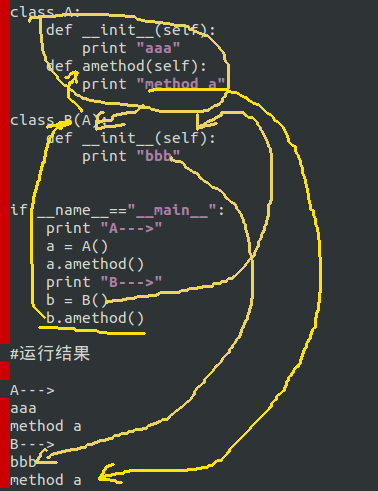## 编写类之四再论继承

``````#!/usr/bin/env python
#coding:utf-8

class Person:
def __init__(self, name, email):
self.name = name
self.email = email

class Programmer(Person):
def __init__(self, name,email,lang, system, website):
Person.__init__(self,name,email)
self.lang = lang
self.system = system
self.website = website

class Pythoner(Programmer):
def __init__(self,name,email):
Programmer.__init__(self,name,email,"python","Ubuntu","qiwsir.github.io")

if __name__=="__main__":
writer = Pythoner("qiwsir","[email protected]")
print "name=",writer.name
print "lang=",writer.lang
print "email=",writer.email
print "system=",writer.system
print "website=",writer.website

#运行结果

name= qiwsir
lang= python
email= [email protected]
system= Ubuntu
website= qiwsir.github.io
``````

## 多余的B

``````#!/usr/bin/env python
#coding:utf-8

class A:
def __init__(self):
print "aaa"

class B(A):
pass

if __name__=="__main__":
a = A()
b = B()

#运行结果

aaa
aaa
``````

B继承A，没有任何修改地继承，B就可以不用写任何东西了，或者说B本质上就是一个多余。在真实的编程过程中，没有这样写的，这里仅仅是为了向看官展示一下继承的含义罢了。

``````##首个继承有效

#!/usr/bin/env python
#coding:utf-8

class A:
def __init__(self):
print "aaa"

class B:
def __init__(self):
print "bbb"

class C1(A,B):
pass

class C2(B,A):
pass

if __name__=="__main__":
print "A--->",
a = A()
print "B--->",
b = B()
print "C1(A,B)--->",
c1 = C1()
print "C2(B,A)--->",
c2 = C2()

#运行结果

A---> aaa
B---> bbb
C1(A,B)---> aaa
C2(B,A)---> bbb
``````

## 其它方法的继承

``````#!/usr/bin/env python
#coding:utf-8

class A:
def __init__(self):
print "aaa"
def amethod(self):
print "method a"

class B(A):
def __init__(self):
print "bbb"

if __name__=="__main__":
print "A--->"
a = A()
a.amethod()
print "B--->"
b = B()
b.amethod()

#运行结果

A--->
aaa
method a
B--->
bbb
method a
``````A的实例和调用，就不多说了。重点看B，类B继承了A，同时，B在构造函数中自己做了规定，也就是B的构造函数是按照B的意愿执行，不执行A的内容，但是，A还有一个amethod(self)方法，B则继承了这个方法。当通过类B的实例调用这个方法的时候，就能够成功了：b.amethod()

``````class A(object):
def method1(self):
print('A.method1')

def method2(self):
print('A.method2')

class B(A):
def method3(self):
print('B.method3')

class C(A):
def method2(self):
print('C.method2')

def method3(self):
print('C.method3')

class D(B, C):
def method4(self):
print('C.method4')

d = D()
d.method4() # 在 D 找到，C.method4
d.method3() # 以 D->B 順序找到，B.method3
d.method2() # 以 D->B->C 順序找到，C.method2
d.method1() # 以 D->B->C->A 順序找到，A.method1
``````